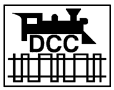HOME > TRAINS > CV Value Calculator for DCCCalculation of CV settings for 4-digit (long) DCC decoder address

Some Digital Command Control (DCC) decoders do not allow direct programming for long (2 byte, 4 digit, extended) addresses. If you need to use paged (or CV) mode, it is necessary to

1. set CV 29 to accept long addresses (usually that means setting bit 5 high)

2. set CV 17 to the high byte and CV1 8 to the low byte of the extended address.

It is cumbersome to calculate those values by hand using a calculator (e.g., the Windows calculator applet) or conversion tables. It is flat out wrong to say that the the first two digits of the 4-digit address are stored in CV 17 and the third and fourth digit of a 4 digit address in CV 18. For example, 1234 is NOT stored as decimal equivalent of 12 in CV 17 and and 34 in CV 18. You need to convert 1234 into a 2-byte, 16 digit binary number, then decode the low byte (left 8 zeros or ones) into the decimal presentation to be set into CV 18, and set the decimal representation of the high byte (first 8 zeros or ones) into CV 17. Complicating is the fact that the first (highest) two digits of the high byte in CV 17 are always set to 1.

Example: for loco address 1234 you need to set CV 17 to 196, and CV 18 to 210

Here you get the answer quickly:

 Enter the desired address and then click the calculate button 4 -digit address         =